# Chapter 11 Partial Credit Models -Part I

In this session, we will learn about
- Formal specifications/parameterisations of the partial credit model
- Estimation of the parameters
- Interpretations of the parameters
- ICCs of the partial credit model
- Recoding data in R

When test items are scored with partial credit scores such as 0, 1 or 2, and not just as correct or incorrect, the model is called the partial credit model (PCM), or the polytomous model (as opposed to the dichotomous model). The dichotomous model is a special case of the partial credit model. So everything included in this section can be applied to the dichotomous model as well.

## 11.1 Advantages of using the Partial Credit Model

If we can separate students’ responses to an item into more than two categories, we obtain more information about the students from this item. For example, instead of saying one group of students are likely of low abilities because they obtained the incorrect answer, and one group are likely of higher abilities because they obtained the correct answer, we can identify students who obtained partially correct answers and deem them likely to be of intermediate abilities. If many items can provide us with more categories than just two scores, the whole test will give us more power to separate students into ability groups reliably based on their test results. That is, the total number of score points of a test is directly related to the reliability of a test.

However, not every item can provide partial scoring. Sometimes an item lends itself only to correct or incorrect answers, such as a Yes/No response. Some items may have low ability students obtaining both incorrect and partially correct answers. In general, when we score items with partial credit scoring, we need to make sure that increasing scores reflect increasing student abilities. In the next chapter, some guidelines to scoring partial credit items are discussed.

## 11.2 Mathematical Parameterisation of the Partial Credit Model

For the dichotomous model (3.1), the probability of success depends on an ability parameter and an item-difficulty parameter. For a partial credit with scores 0, 1 and 2, there are two item-difficult parameters in addition to the ability parameter, as shown below.

$\begin{equation} Prob(X=0) = \frac{1}{1+\exp(\theta - \delta_1)+\exp(2\theta - \delta_1- \delta_2))} \\ \end{equation}$ $\begin{equation} Prob(X=1) = \frac{\exp(\theta - \delta_1)}{1+\exp(\theta - \delta_1)+\exp(2\theta - \delta_1- \delta_2)} \\ \end{equation}$ $\begin{equation} Prob(X=2) = \frac{\exp(2\theta - \delta_1- \delta_2)}{1+\exp(\theta - \delta_1)+\exp(2\theta - \delta_1- \delta_2)}\tag{11.1} \end{equation}$

where $$\theta$$ is the ability parameter, and $$\delta_1$$ and $$\delta_2$$ are two item difficulty parameters for this item. Note that the denominator is just the sum of the numerators, so the probabilities add up to one.

## 11.3 Interpretations of the PCM item difficulty parameters

In the dichotomous case, the item difficulty parameter, $$\delta$$, is the ability measure at which students have 50% chance of obtaining the correct answer for the item. That is, the item difficulty is the ability measure at which the chance of obtaining a 0 or 1 is the same.

In the same way, for the partial credit model, at the $$\delta$$ values, the students have an equal chance of being in two adjacent score categories. For example, students with ability $$\delta_1$$ have an equal chance of obtaining a 0 score or a 1 score. Students with ability $$\delta_2$$ have an equal chance of obtaining a 1 score or a 2 score. Because there are more than 2 score categories, having equal probability of being in two adjacent score categories does not give us all the information about the difficulties of the item overall. The following are graphical examples showing the $$\delta$$ values and possible interpretations.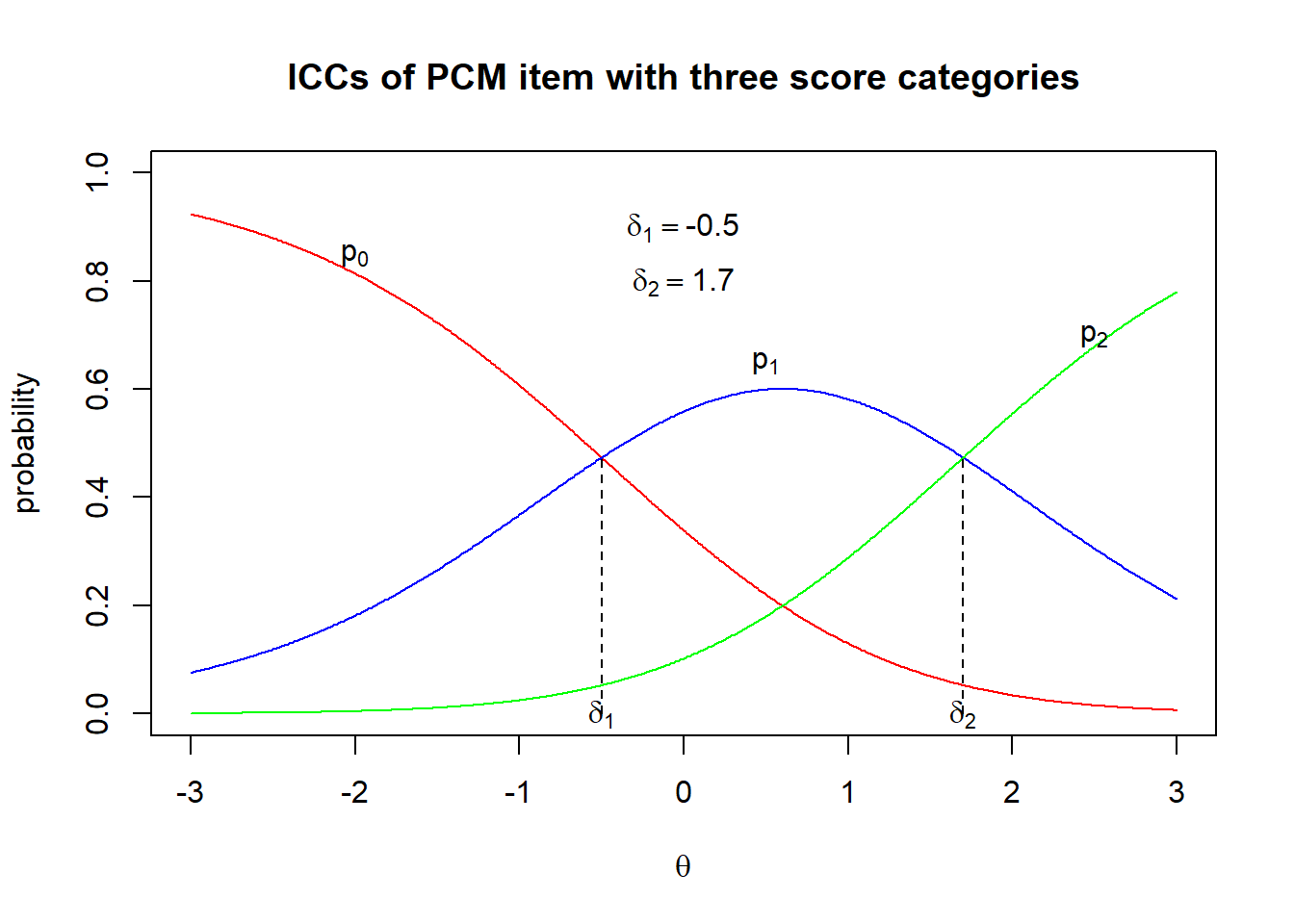Figure 11.1: PCM ICCs with ordered deltas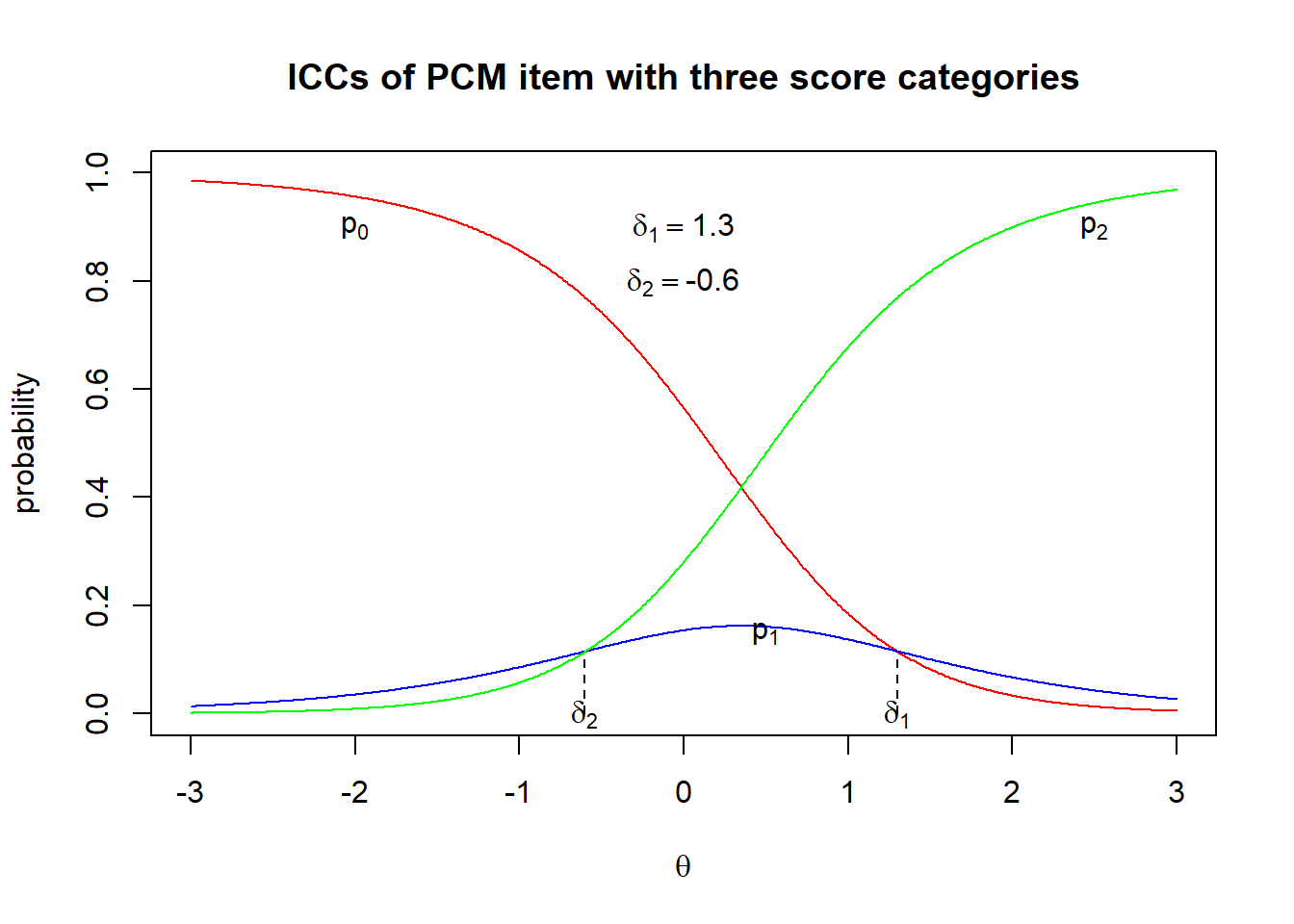Figure 11.2: PCM ICCs with disordered deltas

Figure 11.1 and Figure 11.2 show the item characteristic curves for two PCM items, plotted according to Eq.(11.1).

It can be seen in both Figures that $$\delta_1$$ is the interception point of $$p_0$$ and $$p_1$$, showing the ability at which the two probabilities are equal. Similarly, $$\delta_2$$ is the interception point of $$p_1$$ and $$p_2$$, where the probability of obtaining a score of 1 is the same as the probability of obtaining a score of 2. However, in Figure 11.1, $$\delta_1$$ is smaller than $$\delta_2$$, whereas in Figure 11.2, $$\delta_1$$ is greater than $$\delta_2$$ (known as disordered thresholds). Disordered thresholds occur when the middle score category (score of 1 in this case) has few students as reflected in the low $$p_1$$ curve. Disordered thresholds do not indicate that the item is problematic. It just means that students are most likely to obtain 0 or 2, and few students would obtain a score of 1.

Because the $$\delta$$s can be disordered as in Figure 11.2, the interpretation of the $$\delta$$s is not straightforward. In many cases, the $$\delta$$s should not be regarded as step difficulties of the item, as they reflect only “local” or “conditional” equality of probabilities of two adjacent score categories, while not accounting for the probability of other score categories. More generally, the notion of step difficulties is open to debate.

## 11.4 Thurstonian thresholds

One way to perceive PCM item step difficulties is to use the Thurstonian thresholds. Thurstonian thresholds are defined as the ability at which students have 50% chance of obtaining a score or higher scores. For example, for an item with 0, 1 and 2 scores, the first Thurstonian threshold, $$\gamma_1$$, is the ability at which the students have 50% chance of getting a 1 or 2, as shown below:

$\begin{equation} Prob(X=1 \text{ or } 2/\theta=\gamma_1) = 0.5 \end{equation}$

For the examples in Figure 11.1 and Figure 11.2 above, the Thurstonian thresholds are shown graphically in Figures 11.3 and 11.4.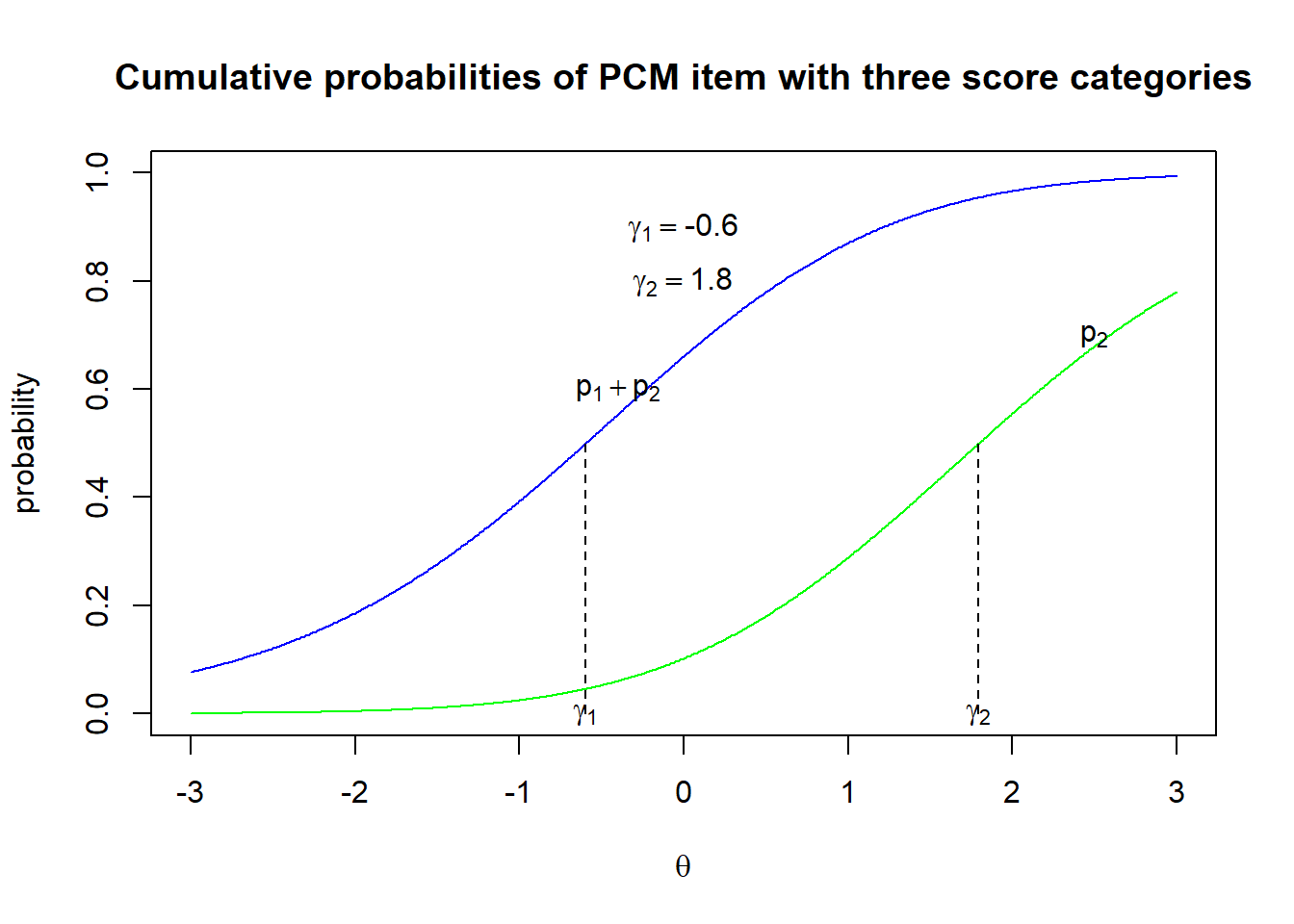Figure 11.3: Thurstonian thresholds Example 1

In Figure 11.3, $$\gamma_1$$ (= -0.6) is the ability at which students have 50% chance of obtaining a score of 1 or 2 (or, at least 1), while $$\gamma_2$$ (= 1.8) is the ability at which students have 50% chance of obtaining a score of 2 (or, at least 2, but 2 is the maximum score in this example). Comparing the deltas with the gammas, where $$\delta_1=$$ -0.5, $$\delta_2=$$ 1.7, and $$\gamma_1=$$ -0.6, $$\gamma_2=$$ 1.8, the differences between deltas and gammas are not very large.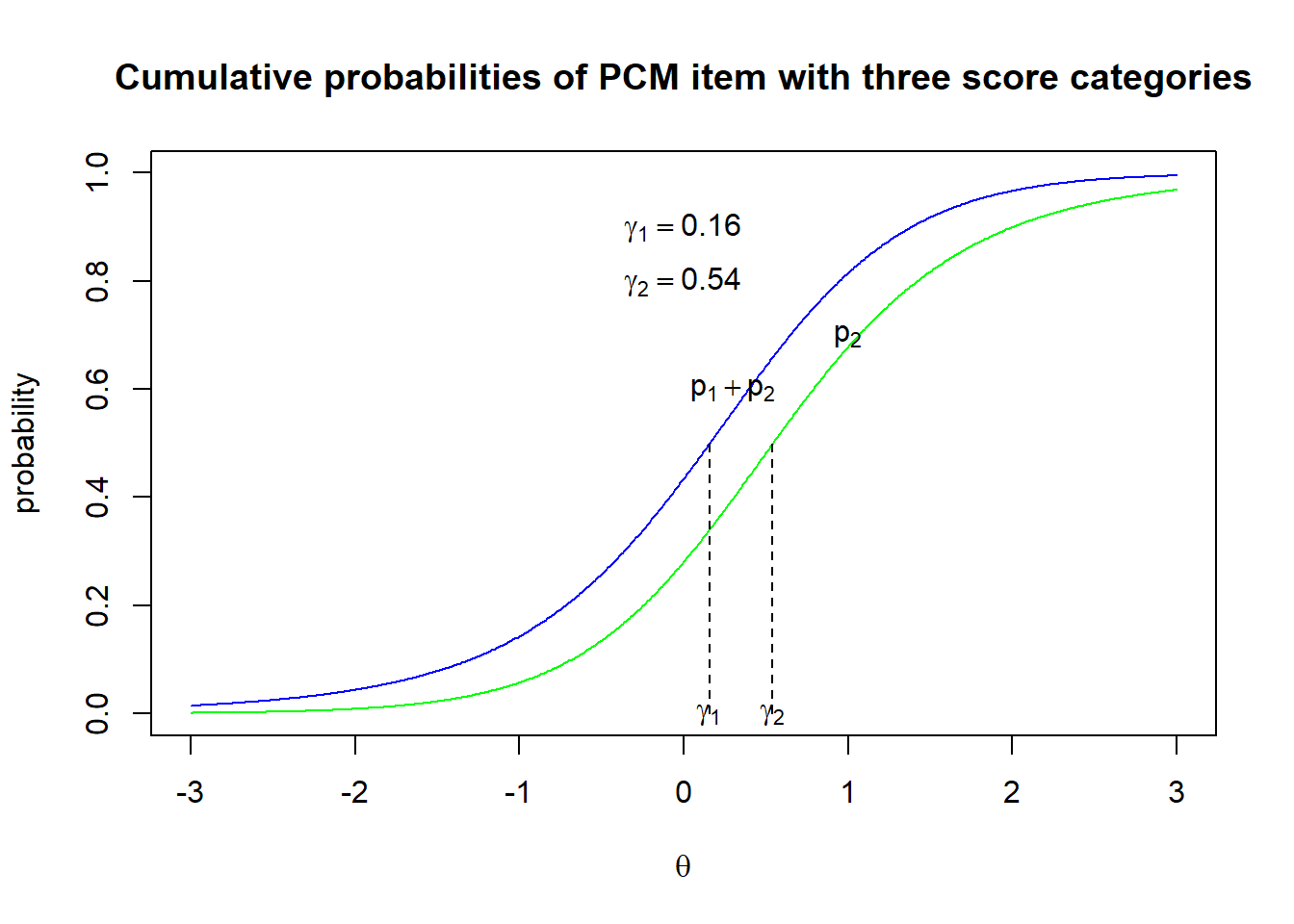Figure 11.4: Thurstonian thresholds Example 2

In Figure 11.4, $$\gamma_1$$ (= 0.16) is the ability at which students have 50% chance of obtaining a score of 1 or 2 (or, at least 1), while $$\gamma_2$$ (= 0.54) is the ability at which students have 50% chance of obtaining a score of 2. Comparing the deltas with the gammas, where $$\delta_1=$$ 1.3, $$\delta_2=$$ -0.6, and $$\gamma_1=$$ 0.16, $$\gamma_2=$$ 0.54, the differences between deltas and gammas are large. The fact that $$\gamma_1$$ is close to $$\gamma_2$$ indicates that few students are in score 1 category, since the probability of getting a score of 1 or 2 is similar to the probability of getting a score of 2, as reflected in the closeness between the $$p_1+p_2$$ curve and the $$p_2$$ curve.

## 11.5 Expected score curve

Another way to think of item difficulty for each score point of a PCM item is to calculate the expected score as a function of ability, and then find the ability at which the expected score is 0, 1, 2, etc.

For example, Figure 11.5 shows the expected score curve.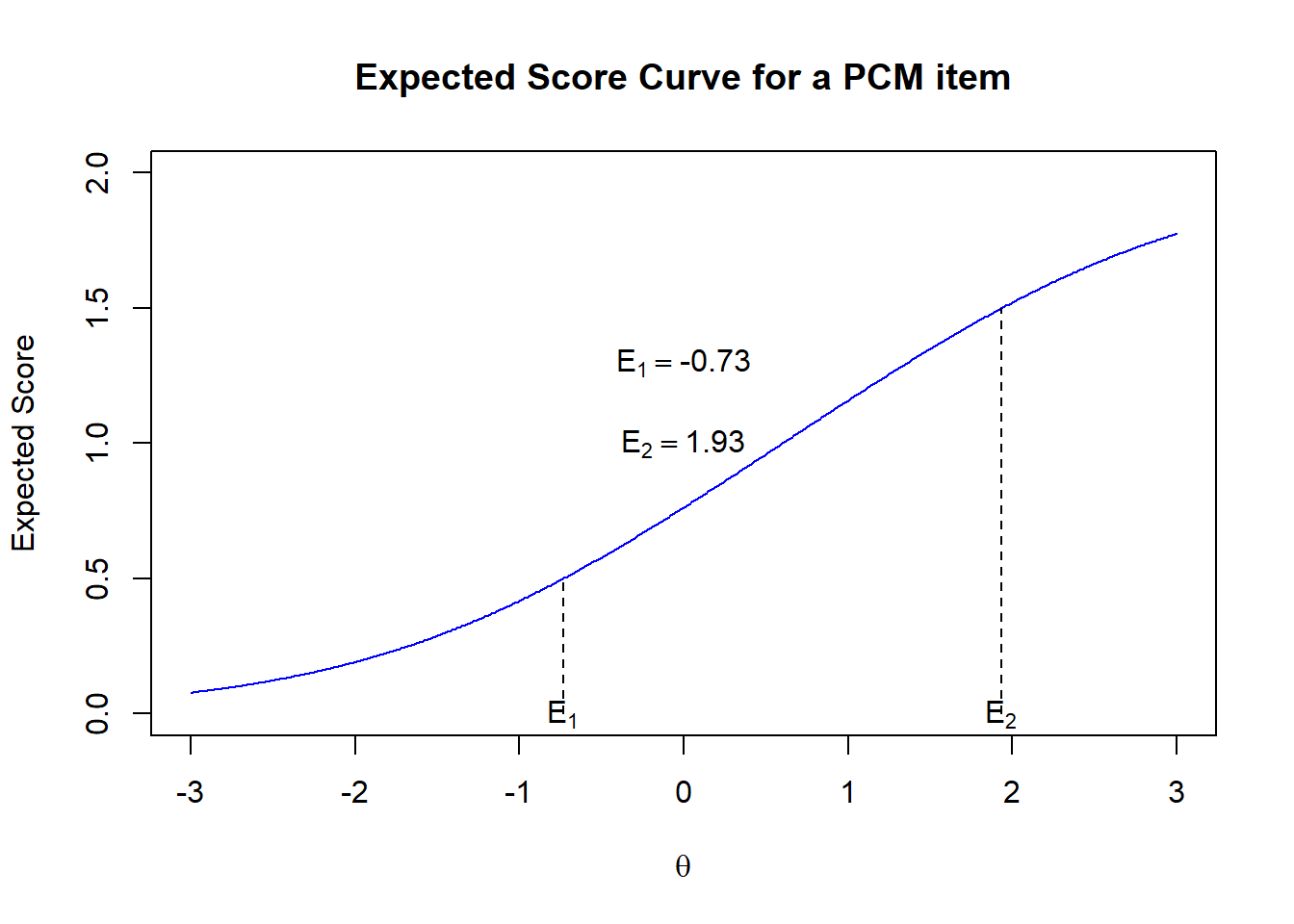Figure 11.5: Expected Score Curve Example 1

Between the ability range of -0.73 and 1.93, the expected score is between 0.5 and 1.5. We may call this ability range the score 1 region. Below -0.73, the expected score is closer to 0 than closer to 1, so this region is the score 0 region. Above an ability of 1.93, the expected score is closer to 2 than closer to 1, so this may be the score 2 region. In this way, -0.73 and 1.93 may be regarded as item difficulty measures for a PCM item. Compare the three sets of potential item difficulty measures: $$\delta_1=$$ -0.5, $$\delta_2=$$ 1.7, $$\gamma_1=$$ -0.6, $$\gamma_2=$$ 1.8, and $$E_1=$$ -0.73, $$E_2=$$ 1.93.

## 11.6 Example R code for fitting a partial credit model

Since the dichotomous model is a special case of the partial credit model, fitting the PCM is the same as fitting the dichotomous model which we have demonstrated in the previous chapters. The data file for this example comes from a survey questionnaire on computer familiarity. Download the files here.

The data file is in text format, coded as A, B, C and D. In the following R code, we will learn about reading in text files and recoding data, as well as producing PCM ICC’s and the Wright Map.

rm(list=ls())
library(TAM)
library(WrightMap)

setwd("C:/G_MWU/ARC/Philippinesfiles")  #set working directory to your folder
resp <- read.fwf("ComputerSurvey.dat", widths=c(rep(1,26)), stringsAsFactors = FALSE)
colnames(resp) <- paste0("Q",seq(1,ncol(resp)))

# recode the data from A, B, C, D to 0, 1, 2, 3, and NA for missing
resp[resp=="A"] <- 0
resp[resp=="B"] <- 1
resp[resp=="C"] <- 2
resp[resp=="D"] <- 3
resp[resp=="Z"] <- NA

#Change data to numeric type
resp <- sapply(resp, as.numeric)
#Find persons with all missing responses
d <- apply(resp,1,function(x){all(is.na(x))})

#remove persons with all missing response
resp <- resp[-which(d),]

mod1 <- tam.jml(resp)

thres <- tam.threshold(mod1) # find Thurstonian thresholds

wrightMap(mod1$WLE, thres, item.side = itemClassic) wrightMap(mod1$WLE, thres)  # try different display options

plot(mod1)  #Expected score curves
plot(mod1,type="items")  #ICCs

mod1\$item1  #show item parameters (deltas)

## 11.7 Exercise

A data set for 20 PCM items, each with three score categories (0, 1 and 2), can be downloaded here. Fit the data to the PCM model. Plot ICCs and Wright Map. Which item(s) is the most difficult? Which item(s) is the easiest?